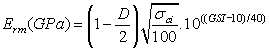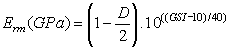RSWebinar: Designing Cost-Effective Ground Improvement Solutions with Settle3 | June 7th, 2023 Register Now

# Parameter Calculator

If you are using the Generalized Hoek-Brown criterion as the strength type for a material, the Parameter Calculator dialog can provide valuable assistance to help you determine the parameters mb, s and a of the Generalized Hoek-Brown criterion. This is very useful because the parameters GSI, mi and D are usually the known quantities for a rock mass, whereas mb, s and a are often not directly available. The rock mass elastic modulus can also be estimated.

To use the Parameter Calculator dialog:

1. In the Define Material Properties dialog, set the Strength Type = Generalized Hoek-Brown.
2. Select the GSI buttonin the Define Material Properties dialog.
3. This will display the Parameter Calculator dialog, which allows you to calculate the parameters mb, s and a, from values of intact rock constant mi, GSI (Geological Strength Index), and Disturbance Factor, D, according to the equations of the Generalized Hoek-Brown criterion.
4. Enter values of GSI, mi, and D in the Parameter Calculator dialog, and the corresponding values of mb, s and a will automatically be displayed in the dialog.
You can use the Pick buttons to assist you in determining appropriate values of GSI, mi and D, see below for details.
1. The checkboxes at the bottom of the dialog allow you to choose whether the calculated values of mb, s and a are applied as the peak and/or residual values. By default, they are both selected.
2. The rock mass elastic modulus will also be estimated if the Compute rock mass elastic modulus check box is selected. See below for details.
3. select [OK] to return to the Material Properties dialog. The calculated values of mb, s and a will automatically be entered as the peak and/or residual strength parameters for the material, according to the selections in step 5. The rock mass elastic modulus will be entered as the Young's Modulus of the material, according to your selections in step 6.

## Pick GSI, mi, D, Intact UCS

Within the Parameter Calculator dialog, you may access interactive tables and charts for:

• the estimation of mi based on rock type,
• the estimation of GSI based on rock structure and surface conditions,
• the estimation of Disturbance Factor based on excavation type and techniques,
• the estimation of Intact Uniaxial Compressive Strength (UCS) based on field estimates.

Select the Pickbutton for the parameter that you wish to estimate, and you will see a table or chart which allows you to select an appropriate value for the parameter, for your material.

## Rock mass elastic modulus

In the Parameter Calculator dialog, the rock mass elastic modulus will be automatically calculated if the Compute rock mass elastic modulus check box is selected.

The elastic modulus of a rock mass is often not a well-known or easily measured parameter. Several researchers have proposed empirical relationships for estimating the rock mass modulus on the basis of classification schemes. The methods available in RS2 are based on the GSI rock mass classification scheme and the Generalized Hoek-Brown strength criterion.

Three calculation methods are available:

• Generalized Hoek-Diederichs
• Simplified Hoek-Diederichs
• Hoek, Carranza-Torres, Corkum

The methods are based on the equations given below.

The Generalized Hoek-Diederichs method is the default and recommended modulus estimation method. The other two methods are available for comparative purposes, but their use is no longer recommended.

### Generalized Hoek-Diederichs

The generalized Hoek and Diederichs equation (Hoek & Diederichs, 2006) utilizes the intact rock modulus Ei, GSI and D to compute the rock mass modulus Erm. Equation 1 assumes MPa units. If you are using a different unit system an appropriate conversion is applied.Eqn.1

If you choose this method, then you must enter the intact rock modulus Ei. You can enter the intact rock modulus directly, or you can enter the modulus ratio (MR). Intact modulus (Ei) is equal to the modulus ratio (MR) multiplied by the uniaxial compressive strength of the intact rock (sigci).

Ei = MR (sigci) Eqn.2

If you are using the modulus ratio (MR) option, then the value of Ei is automatically calculated using the current values of MR and sigci entered in the dialog (e.g. if you change sigci or MR, then Ei will automatically be re-calculated according to Equation 2).

NOTE: you can select the Pickbutton beside the MR edit box to estimate the modulus ratio according to rock type.

### Simplified Hoek-Diederichs

The Simplified Hoek & Diederichs equation for rock mass modulus, (Hoek & Diederichs, 2006), only requires GSI and D (Disturbance Factor) as input parameters. Equation 3 assumes MPa units. If you are using a different unit system an appropriate conversion is applied.Eqn.3

This equation can be used if reliable values of intact rock modulus are NOT available.

### Hoek, Carranza-Torres, Corkum

The Hoek, Carranza-Torres and Corkum equation for rock mass modulus (Hoek, Carranza-Torres and Corkum, 2002), uses the uniaxial compressive strength of the intact rock (sigci), GSI and D.

For sigci <= 100 MPa, Equation 4 is used:Eqn.4

For sigci > 100 MPa, Equation 5 is used:Equations 4 and 5 assume GPa units. If you are using a different unit system an appropriate conversion is applied.